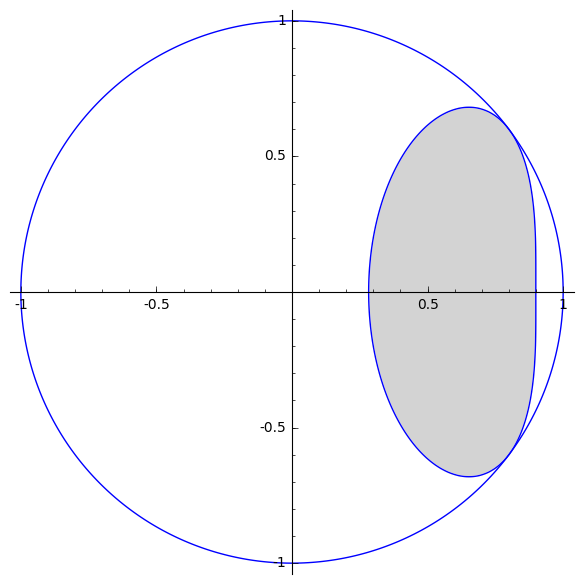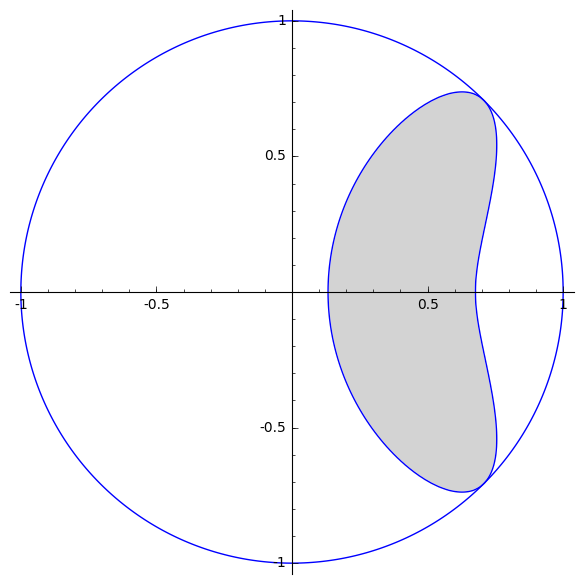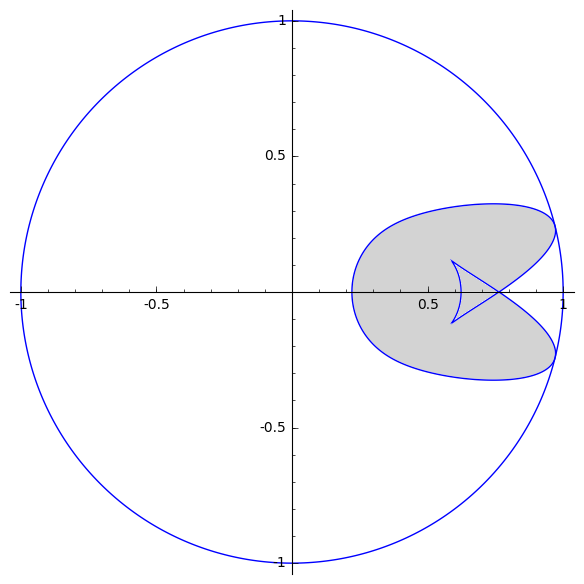# Normalized numerical range

## Definition

The classical numerical range $F(A)$ of $A\in M_n(\mathbb{C})$ is by definition the set of values of the corresponding quadratic form $x^*Ax$ on the unit sphere $S^n := \{x ^n : \|x \|=1 \}$of$^n$. Equivalently, $F(A) =\{(x^*Ax)/||x||2 xn\{0\}\}.$ Various modifications and generalization of the numerical range have been considered in the literature. Our paper is concerned with the so called normalized numerical range. Defined as $F_N(A) := \{ x ^n, Ax 0 \},$ it was introduced in , and then further investigated in for example .

## Properties

Suppose that $A \in M_n(\mathbb{C})$. Then:

• For all $z \in F_N(A)$, $|z| \le 1$.

*If $z \in F_N(A)$, then $|z| = 1$ if and only if $z = /||$for some$(A)$.

• $F_N(A)$ is unitarily invariant: $F_N(U^*AU)=F_N(A)$ for any unitary $U\in M_n(\mathbb{C})$.

• $F_N(e^{i\theta}A) = e^{i\theta} F_N(A)$ for all $[0,2)$.

• $F_N(cA) = F_N(A)$ for all $c > 0$.

• If $A$ is invertible, then $F_N(A)$ is closed.

The following theorems and examples are taken from .

## Theorem 1

Suppose that $A \in M_2(\mathbb{C})$ has non-zero eigenvalues $\lambda_1, \lambda_2$ such that $\lambda_1/\lambda_2 < 0$. Then $F_N(A)$ is a closed elliptical disk. In the case when $A > 0$, the ellipse is given by the equation

## Theorem 2

For $A \in M_2 (\mathbb{C}) \backslash \{0\}$ with eigenvalues $\lambda_1$ and $\lambda_2$, the boundary of $F_N(A)$ is an ellipse if and only if $|\lambda_1| = |\lambda_2|$ or $\lambda_1/\lambda_2 < 0$.

### Examples

1. The normalized numerical range of $A =$.2. The normalized numerical range of $B =$.3. The normalized numerical range of $C =$.For matrix $A$ is a typical example of convex normalized numerical range that is not an ellipse. The normalized numerical range of matrix $B$ is not convex, but has a smooth boundary (boundary is differentiable). Finally, the last example for matrix $C$ has a boundary that is not smooth at one point. All three of these examples have boundaries that satisfy irreducible 8th degree polynomial equations.

1. W. Auzinger, “Sectorial operators and normalized numerical range,” Applied numerical mathematics, vol. 45, no. 4, pp. 367–388, 2003, [Online]. Available at: https://www.sciencedirect.com/science/article/pii/S0168927402002544.
2. L. Z. Gevorgyan, “Normalized numerical ranges of some operators,” Operators and Matrices, vol. 3, no. 1, pp. 145–153, 2009, [Online]. Available at: https://nyuscholars.nyu.edu/en/publications/on-the-normalized-numerical-range.
3. B. Lins, I. M. Spitkovsky, and S. Zhong, “The normalized numerical range and the Davis–Wielandt shell,” Linear Algebra and its Applications, vol. 546, pp. 187–209, 2018, [Online]. Available at: https://www.sciencedirect.com/science/article/pii/S0024379518300417.